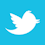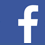Commonly available tools can determine the relationship between gold thickness and plating time.

Regression analysis utilizes the relationship between quantitative variables to predict one variable from one or more other variables. These relationships are either functional or statistical. Functional relationships have perfect fits. For example, ice cream cones cost \$1 each, so one can buy 10 ice cream cones for \$10 or 20 ice cream cones for \$20. Statistical relationships typically do not have perfect fits. For example, cholesterol levels and body weight have a non-perfect fit. It is commonly accepted that the term “regression” describes the statistical, not the functional, relationship between variables.1

A regression model is a formal means of expressing the general tendency of a dependent variable (Y) to vary with the independent variable (X) systematically. The independent variable (X) is also referred to as the predictor variable. George Box (2007) states, “all models are approximations. Essentially, all models are wrong, but some are useful. However, the approximate nature of the model must always be borne in mind” (p. 414), implying there are random errors present, hence the nonperfect fit.1,2 The expression of the general tendency of a dependent variable (Y) and an independent variable (X) is potent in many different fields.